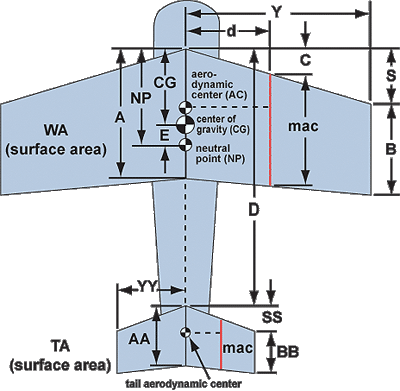A i r c r a f t   S u p e r   C a l c u l a t o r    6 . 1
(Mean Aerodynamic Chord, Neutral Point, Center of Gravity, and Weight & Balance)

MAC And Neutral Point
If no changes are seen in results after updating an input field,
then click BOTH compute/refresh buttons.

use the same units of measure (inches or feet) for all entries!Center of Gravity (CG) is the point where the WEIGHT of the aircraft is balanced.  Neutral Point (NP) is the point where the AERODYNAMIC FORCES generated by the wing and tail are balanced. Placing CG 5% - 15% of MAC in front of NP creates a longitudinal (pitch) stability called Static Margin. A lower margin produces less stability and greater elevator authority, while a higher margin creates more stability and less elevator authority. Too much static margin results in elevator stall at take off and landing. 25% - 35% MAC is generally accepted as a good range for the CG of a conventional tailed aircraft where the AC of the wing is at 25% MAC.

The Equations

 Wing Sweep, C = (S * (A + 2B)) / (3 * (A + B)) MAC (length) = A - (2 * (A - B) * (0.5 * A + B) / (3 * (A + B))) MAC location, d = Y * ((A - MAC) / (A - B)) wing area, WA = Y * (A + B) tail area, TA = YY * (AA + BB) wing aspect ratio, ARw = ((Y * 2) ^ 2) / WA tail aspect ratio, ARt = ((YY * 2) ^ 2) / TA Tail Arm = (D - wing AC) + tail AC tail volume, Vbar = (TA / WA) * (Tail Arm / MAC) NP (%MAC) = 0.25 + (0.25 * sqr(sqr(ARw)) * Vbar ideal CG (%MAC) = NP - Desired Static Margin Moment (total) = (Lt wheel Wt * Lt Arm) + (Rt Wheel Wt * Rt Arm) + (Center Wheel Wt * Center Arm) + (Pilot Wt * Pilot Arm) + ( Psngr Wt * Psngr Arm) + (Fuel Wt * Fuel Arm) actual CG (%MAC) = ((Moment / Total Wt) - (Datum2LE + C)) / MAC actual static margin % = (NP - actual CG) / MAC * 100

 NOTE:   This calculator can be distributed by permission only simply by copying the contents between the first and last tags.  Javascript equations and functions are fully contained within it.  DO NOT REPURPOSE BY EDITING any html or javascript contained herein without permission. email Dean A. Scott at dascott / at / southern / dot / edu to obtain distribution clearance and repurposing/reuse permission.       CG / MAC Calculator adapted from the Public Domain. Neutral Point and Weight & Balance Calculators developed by Dean A. Scott (c) 2005, all rights reserved.  Use with permission. NP equations courtesy Alasdair Sutherland (see C.G. Position article on Bloobird Radio Flyers)

Enter the following data:

Wing
Tail
Root Chord (A, AA) :
Tip Chord (B, BB) :
Sweep Distance (S, SS) :
Half Span (Y, YY) :
Distance between wing & tail root LE's (D) :
Desired Static Margin
(5% = twitchy  20% = mushy  10% - 15% recommended) :

Weight And Balance

Weight
Arm
Left Main Wheel :
Right Main Wheel :
Nose or Tail Wheel :
Pilot :
Passenger :
Fuel Tank (gallons of gas) :

Datum Point from Wing Root LE :
Wing Root Chord (A) :
Sweep length at MAC :
MAC length :
For more accurate results, enter the values for Sweep Length and MAC
computed from the "MAC and NP" calculator above (shown below in yellow).

Calculated Results
Mean Aerodynamic Chord (MAC) =
MAC distance from root chord (d) =
Wing Aerodynamic Center aft of root LE =
Ideal Center of Gravity %MAC =
Actual Center of Gravity %MAC =
Ideal CG aft of root LE =
Actual CG aft of root LE =
Actual CG from main axle (neg. is aft) =
Neutral Point %MAC (NP) =
Neutral Point aft of Wing's root LE (NP) =
Distance between Ideal CG and NP (E) =
Distance between Actual CG and NP =
Desired Static Margin % =
Actual Static Margin % =
Gross Takeoff Weight of Aircraft =
Empty Weight of Aircraft =

Other useful results

Wing Area =
Tail Area =
Wing Apsect =
Tail Aspect =
Wing Taper =
Tail Taper =
W sweep C =
T sweep CC =
Tail MAC =
T MAC dist =
Tail Arm =
Vbar =
Lt wheel mnt =
Rt wheel mnt =
Center wheel mnt =
Pilot mnt =
Passngr mnt =
Tank mnt =